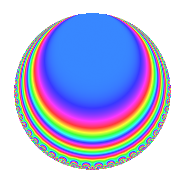# Properties

 Label 1728.2.c.cLevel 1728 Weight 2 Character orbit 1728.c Analytic conductor 13.798 Analytic rank 0 Dimension 4 CM no Inner twists 4

# Related objects

## Newspace parameters

 Level: $$N$$ $$=$$ $$1728 = 2^{6} \cdot 3^{3}$$ Weight: $$k$$ $$=$$ $$2$$ Character orbit: $$[\chi]$$ $$=$$ 1728.c (of order $$2$$, degree $$1$$, not minimal)

## Newform invariants

 Self dual: no Analytic conductor: $$13.7981494693$$ Analytic rank: $$0$$ Dimension: $$4$$ Coefficient field: $$\Q(\sqrt{3}, \sqrt{-5})$$ Coefficient ring: $$\Z[a_1, \ldots, a_{11}]$$ Coefficient ring index: $$2^{2}$$ Twist minimal: no (minimal twist has level 108) Sato-Tate group: $\mathrm{SU}(2)[C_{2}]$

## $q$-expansion

Coefficients of the $$q$$-expansion are expressed in terms of a basis $$1,\beta_1,\beta_2,\beta_3$$ for the coefficient ring described below. We also show the integral $$q$$-expansion of the trace form.

 $$f(q)$$ $$=$$ $$q -\beta_{1} q^{5} -\beta_{2} q^{7} +O(q^{10})$$ $$q -\beta_{1} q^{5} -\beta_{2} q^{7} -\beta_{3} q^{11} -2 q^{13} -2 \beta_{1} q^{17} -4 \beta_{3} q^{23} + 2 \beta_{1} q^{29} + \beta_{2} q^{31} -5 \beta_{3} q^{35} + 4 q^{37} + 4 \beta_{1} q^{41} -2 \beta_{2} q^{43} -2 \beta_{3} q^{47} -8 q^{49} -\beta_{1} q^{53} + \beta_{2} q^{55} -2 \beta_{3} q^{59} + 4 q^{61} + 2 \beta_{1} q^{65} + 2 \beta_{2} q^{67} -6 \beta_{3} q^{71} + 5 q^{73} + 3 \beta_{1} q^{77} + 2 \beta_{2} q^{79} -7 \beta_{3} q^{83} -10 q^{85} -2 \beta_{1} q^{89} + 2 \beta_{2} q^{91} + 11 q^{97} +O(q^{100})$$ $$\operatorname{Tr}(f)(q)$$ $$=$$ $$4q + O(q^{10})$$ $$4q - 8q^{13} + 16q^{37} - 32q^{49} + 16q^{61} + 20q^{73} - 40q^{85} + 44q^{97} + O(q^{100})$$

Basis of coefficient ring in terms of a root $$\nu$$ of $$x^{4} + x^{2} + 4$$:

 $$\beta_{0}$$ $$=$$ $$1$$ $$\beta_{1}$$ $$=$$ $$($$$$\nu^{3} + 3 \nu$$$$)/2$$ $$\beta_{2}$$ $$=$$ $$2 \nu^{2} + 1$$ $$\beta_{3}$$ $$=$$ $$($$$$-\nu^{3} + \nu$$$$)/2$$
 $$1$$ $$=$$ $$\beta_0$$ $$\nu$$ $$=$$ $$($$$$\beta_{3} + \beta_{1}$$$$)/2$$ $$\nu^{2}$$ $$=$$ $$($$$$\beta_{2} - 1$$$$)/2$$ $$\nu^{3}$$ $$=$$ $$($$$$-3 \beta_{3} + \beta_{1}$$$$)/2$$

## Character values

We give the values of $$\chi$$ on generators for $$\left(\mathbb{Z}/1728\mathbb{Z}\right)^\times$$.

 $$n$$ $$325$$ $$703$$ $$1217$$ $$\chi(n)$$ $$1$$ $$-1$$ $$-1$$

## Embeddings

For each embedding $$\iota_m$$ of the coefficient field, the values $$\iota_m(a_n)$$ are shown below.

For more information on an embedded modular form you can click on its label.

Label $$\iota_m(\nu)$$ $$a_{2}$$ $$a_{3}$$ $$a_{4}$$ $$a_{5}$$ $$a_{6}$$ $$a_{7}$$ $$a_{8}$$ $$a_{9}$$ $$a_{10}$$
1727.1
 0.866025 + 1.11803i −0.866025 + 1.11803i −0.866025 − 1.11803i 0.866025 − 1.11803i
0 0 0 2.23607i 0 3.87298i 0 0 0
1727.2 0 0 0 2.23607i 0 3.87298i 0 0 0
1727.3 0 0 0 2.23607i 0 3.87298i 0 0 0
1727.4 0 0 0 2.23607i 0 3.87298i 0 0 0
 $$n$$: e.g. 2-40 or 990-1000 Significant digits: Format: Complex embeddings Normalized embeddings Satake parameters Satake angles

## Inner twists

Char Parity Ord Mult Type
1.a even 1 1 trivial
3.b odd 2 1 inner
4.b odd 2 1 inner
12.b even 2 1 inner

## Twists

By twisting character orbit
Char Parity Ord Mult Type Twist Min Dim
1.a even 1 1 trivial 1728.2.c.c 4
3.b odd 2 1 inner 1728.2.c.c 4
4.b odd 2 1 inner 1728.2.c.c 4
8.b even 2 1 108.2.b.a 4
8.d odd 2 1 108.2.b.a 4
12.b even 2 1 inner 1728.2.c.c 4
24.f even 2 1 108.2.b.a 4
24.h odd 2 1 108.2.b.a 4
72.j odd 6 2 324.2.h.d 8
72.l even 6 2 324.2.h.d 8
72.n even 6 2 324.2.h.d 8
72.p odd 6 2 324.2.h.d 8

By twisted newform orbit
Twist Min Dim Char Parity Ord Mult Type
108.2.b.a 4 8.b even 2 1
108.2.b.a 4 8.d odd 2 1
108.2.b.a 4 24.f even 2 1
108.2.b.a 4 24.h odd 2 1
324.2.h.d 8 72.j odd 6 2
324.2.h.d 8 72.l even 6 2
324.2.h.d 8 72.n even 6 2
324.2.h.d 8 72.p odd 6 2
1728.2.c.c 4 1.a even 1 1 trivial
1728.2.c.c 4 3.b odd 2 1 inner
1728.2.c.c 4 4.b odd 2 1 inner
1728.2.c.c 4 12.b even 2 1 inner

## Hecke kernels

This newform subspace can be constructed as the intersection of the kernels of the following linear operators acting on $$S_{2}^{\mathrm{new}}(1728, [\chi])$$:

 $$T_{5}^{2} + 5$$ $$T_{7}^{2} + 15$$

## Hecke characteristic polynomials

$p$ $F_p(T)$
$2$ 1
$3$ 1
$5$ $$( 1 - 5 T^{2} + 25 T^{4} )^{2}$$
$7$ $$( 1 + T^{2} + 49 T^{4} )^{2}$$
$11$ $$( 1 + 19 T^{2} + 121 T^{4} )^{2}$$
$13$ $$( 1 + 2 T + 13 T^{2} )^{4}$$
$17$ $$( 1 - 14 T^{2} + 289 T^{4} )^{2}$$
$19$ $$( 1 - 19 T^{2} )^{4}$$
$23$ $$( 1 - 2 T^{2} + 529 T^{4} )^{2}$$
$29$ $$( 1 - 38 T^{2} + 841 T^{4} )^{2}$$
$31$ $$( 1 - 47 T^{2} + 961 T^{4} )^{2}$$
$37$ $$( 1 - 4 T + 37 T^{2} )^{4}$$
$41$ $$( 1 - 2 T^{2} + 1681 T^{4} )^{2}$$
$43$ $$( 1 - 26 T^{2} + 1849 T^{4} )^{2}$$
$47$ $$( 1 + 82 T^{2} + 2209 T^{4} )^{2}$$
$53$ $$( 1 - 101 T^{2} + 2809 T^{4} )^{2}$$
$59$ $$( 1 + 106 T^{2} + 3481 T^{4} )^{2}$$
$61$ $$( 1 - 4 T + 61 T^{2} )^{4}$$
$67$ $$( 1 - 74 T^{2} + 4489 T^{4} )^{2}$$
$71$ $$( 1 + 34 T^{2} + 5041 T^{4} )^{2}$$
$73$ $$( 1 - 5 T + 73 T^{2} )^{4}$$
$79$ $$( 1 - 16 T + 79 T^{2} )^{2}( 1 + 16 T + 79 T^{2} )^{2}$$
$83$ $$( 1 + 19 T^{2} + 6889 T^{4} )^{2}$$
$89$ $$( 1 - 158 T^{2} + 7921 T^{4} )^{2}$$
$97$ $$( 1 - 11 T + 97 T^{2} )^{4}$$